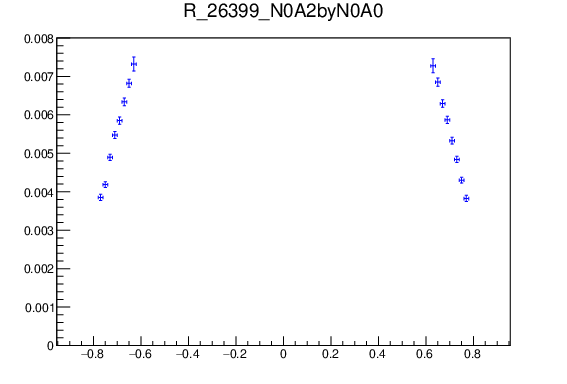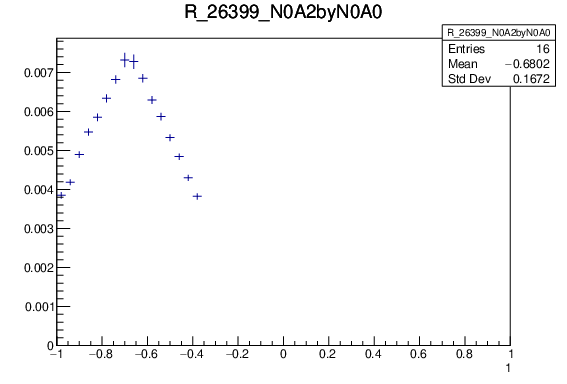# Issue when converting TGraph to TH1

When I convert a TGraph to a TH1 it is not ignoring all the empty bins, and seems to push everything together into the wrong set of bins. The first image is what I have before conversion, and the second image after (both plots should look the same):``````for (int i=0; i<12; i++){ //Loop over all the histograms

h = new TH1D (Form("%s", Ratio_Plot_Names[i].c_str()), Form("%s", Ratio_Plot_Names[i].c_str()), 50, -1.0, 1.0);

TGraphAsymmErrors *graph = (TGraphAsymmErrors*)File->Get(Ratio_Plot_Names[i].c_str() );
TCanvas *c1 = new TCanvas (Form("c%i", i),Form("c%i",i), 700, 500);

int nPoints = graph->GetN(); //Number of points in the TGraph

for (int j=0; j < nPoints; j++){ //Loop through the points in the TGraph

double x,y, ex, ey;
graph->GetPoint(j,x,y);
ex = graph->GetErrorX(j);
ey = graph->GetErrorY(j);

h->SetBinContent(j+1,y);
h->SetBinError(j+1,ey);

}//Closing loop over TGraph points (j)

h->Draw();
``````

I’m not sure how to resolve this, or why it’s even doing it like that.

ROOT Version: Not Provided
Platform: Not Provided
Compiler: Not Provided

you are mixing bin number and position (index) in the graph.

Try with:

``````    Int_t k = h->FindFixBin(x); // ... or ... (x + h->GetBinWidth(1) / 2.0)
h->SetBinContent(k, y);
h->SetBinError(k, ey);
``````

This solves the problem. However, for other variables where there are no empty bins, then they come out all funny. So I’ve implemented this as an ‘else if’ statement for the variable shown. Thank you!

This topic was automatically closed 14 days after the last reply. New replies are no longer allowed.